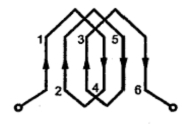### Types of Armature Winding

We have seen that there are number of armature conductors, which are connected in specific manner as per the requirements, which is called armature winding. According to the way of connecting the conductors, armature winding has basically two types namely,
a) Lap winding          b) Wave winding

1.1 Lap winding
In this case, if connection is started from conductor in slot 1 then connections overlap each other as winding proceeds, till starting point is reached again.
Developed view of part of the armature winding in lap fashion shown in the Fig. 1.Fig. 1 Lap Winding

As seen from the Fig. 1, there is overlapping of coils while proceeding.
Note : Due to such connection, the total number of conductors get divided into 'P' number of parallel paths, where P = number of pole sin the machine.
Large number of parallel paths indicate high current capacity machine hence lap winding is preferred for high current rating generators.
1.2 Wave Winding
In this type of connection, winding always travels ahead avoiding overlapping. It travel like a progressive wave hence called wave winding. To get an idea of wave winding a part of armature winding in wave fashion is shown in the Fig. 2.Fig. 2 Wave winding

Both coils starting from slot 1 and slot 2 are progressing in wave fashion.
Note : Due to this type of connection, the total number of conductors get divided into two number of parallel paths always, irrespective of number of poles of the machine. As number of parallel paths are less, it is preferrable for low current, high voltage capacity generators.
The number of parallel paths in which armature conductors are divided due to lap or wave fashion of connection is denoted as A. So A = P for lap connection and A = 2 for wave connection.
1.3 Comparison of Lap and Wave Type Winding